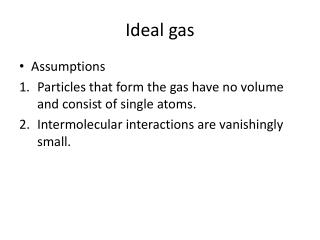# Ideal gas - PowerPoint PPT PresentationDownload PresentationIdeal gas

Ideal gas
Download Presentation## Ideal gas

- - - - - - - - - - - - - - - - - - - - - - - - - - - E N D - - - - - - - - - - - - - - - - - - - - - - - - - - -
##### Presentation Transcript

1. Ideal gas • Assumptions • Particles that form the gas have no volume and consist of single atoms. • Intermolecular interactions are vanishingly small.

2. Ideal gas Equations of state PV=NkT P= pressure V= volume N=number of particles of gas k= Boltzmann Constant= 1.38x10-23J/K K=Kelvin temperature

3. Ideal gas Equations of state PV=nRT P= pressure V= volume n=number of moles of gas R= Universal Gas Constant= K=Kelvin temperature

5. Ideal gas Relationship between Avogadro’s number, Universal Gas constant, and Boltzmann constant.

6. Kinetic –molecular theory • Many molecules are in a container and they behave like point particles.(No volume) • The molecules move around randomly, and obey Newton’s laws. • The only interactions that the molecules undergo are elastic collisions with each other and the walls of the container.

7. Kinetic –molecular theory Pressure is a result of the molecules colliding with the walls of the container. As the number of molecules or thir average speed increases, the pressure increases.

8. Kinetic –molecular theory Results of kinetic-molecular theory.

9. Kinetic –molecular theory Results of kinetic-molecular theory.

10. Kinetic –molecular theory Internal energy of an ideal monatomic gas..

11. Kinetic –molecular theory Other gas laws – the amount of gas does not change

12. Laws of Thermodynamics The first Law of Termodynamics – If U is the internal energy of a system, than DU=Q-W. If Q>0 System gains heat If Q<0 System loses heat If W>0 Work is done by the system If W<0 Work is done on the system

13. Laws of Thermodynamics The first Law of Thermodynamics – If U is the internal energy of a system, than DU=Q-W

14. Table 18-1Signs of Q and W

15. Figure 18-1The Internal Energy of a System

16. Figure 18-2Work and Internal Energy

17. Laws of Thermodynamics At constant pressure, the work done by or on a system is W=PΔV The area under a PV curve represents work. If a process occurs at a constant volume, the work done during the process is 0.

18. Figure 18-5A Constant-Pressure Process

19. Example 18-2Work Area

20. Laws of Thermodynamics Isothermal processes – these are processes that take place at a constant temperature. PV=constant

21. Figure 18-8Isotherms on a PV Plot

22. Laws of Thermodynamics Adiabatic processes – these are processes that take place without heat entering or leaving the system.

23. Figure 18-9An Isothermal Expansion